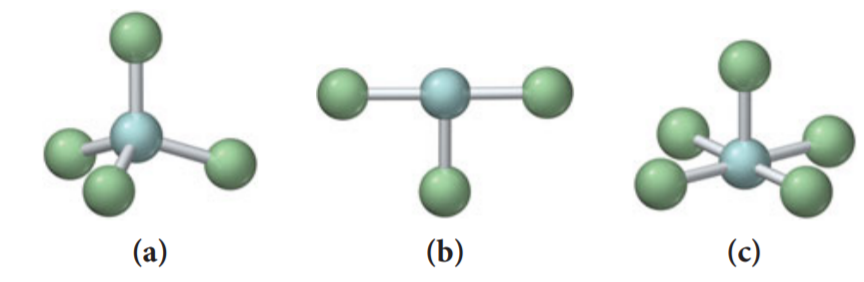×
Get Full Access to Chemistry: A Molecular Approach - 3 Edition - Chapter 10 - Problem 33e
Get Full Access to Chemistry: A Molecular Approach - 3 Edition - Chapter 10 - Problem 33e

×

Solved: For each molecular geometry, list the number ofISBN: 9780321809247 1

Solution for problem 33E Chapter 10

Chemistry: A Molecular Approach | 3rd Edition

• Textbook Solutions
• 2901 Step-by-step solutions solved by professors and subject experts
• Get 24/7 help from StudySoup virtual teaching assistantsChemistry: A Molecular Approach | 3rd Edition

4 5 1 366 Reviews
15
0
Problem 33E

For each molecular geometry, list the number of total electron groups, the number of bonding groups, and the number of lone pairs on the central atom.Step-by-Step Solution:

Solution :Step 1 : Here , we will be using ideal gas equation to fill the data in the table.Let’s consider the 1st row of data : Here, we are given, ‘P’, ‘V’ and ‘n’, we should calculate ‘T’ :P = 2.00 atm , V = 1.00 L , n = 0.500 mol, T = (T should be found in K)Consider the ideal gas equation : PV = nRT T = nR T = 48.72 K

Step 2 of 5

Step 3 of 5

ISBN: 9780321809247

Unlock Textbook Solution